5.1.3. Thermal bremsstrahlung from intracluster gas

Felten et al. (1966) first suggested that the X-ray emission from clusters (the Coma cluster in particular) was due to diffuse intracluster gas at a temperature of Tg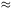108 K and an atomic density of n10-3 cm-3. (Unfortunately, the early X-ray detection of Coma that they sought to explain was spurious.) At such temperatures and densities, the primary emission process for a gas composed mainly of hydrogen is thermal bremsstrahlung (free-free) emission. The emissivity at a frequencyof an ion of charge z in a plasma with an electron temperature Tg is given by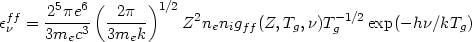(5.11)

where ni and ne are the number density of ions and electrons, respectively. The emissivity is defined as the emitted energy per unit time, frequency, and volume V,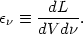(5.12)

The Gaunt factor gff(Z, Tg,) corrects for quantum mechanical effects and for the effect of distant collisions, and is a slowly varying function of frequency and temperature given in Karzas and Latter (1961) and Kellogg et al. (1975). If the intracluster gas is mainly at a single temperature, then equation (5.11) indicates that the X-ray spectrum should be close to an exponential of the frequency. In fact, the observed X-ray spectra are generally fit fairly well by this equation (Section 4.3.1), with gas temperatures of 2 × 107 to 108 K. This equation predicts that the emission from clusters should fall off rapidly at high frequencies, as is observed.

As first noted by Felten et al. (1966), if the intracluster gas either came out of galaxies or fell into the cluster from intergalactic space, it would have a temperature such that the typical atomic velocity was similar to the velocity of galaxies in the cluster. That is,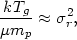(5.13)

where µ is the mean molecular weight in amu, mp is the proton mass, andr is the line-of-sight velocity dispersion of galaxies in the cluster. If the gas came out of galaxies, it would have the same energy per unit mass as the matter in galaxies. If it fell into clusters, it has roughly the same velocity dispersion because it responds to the same gravitational potential as the galaxies. Finally, if the gas were heated so that its initial energy per mass were much greater than that given by equation (5.13), it would not be bound to the cluster and would escape as a wind. Even in this situation, most of the extra energy would go into the kinetic energy of the wind (Section 5.6), and the gas temperature would stay within a factor of 3 or so of equation (5.13). Thus this model predicts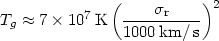(5.14)

for a solar abundance plasma, in reasonable agreement with the temperatures required to explain the X-ray spectra (see equation 4.10).

If the intracluster gas is in hydrostatic equilibrium in the cluster potential, and if the gas temperature is given roughly by equation (5.14), then the spatial distribution of the gas and galaxies will be similar. In fact, the observed X-ray surface brightness of clusters is similar to the projected distribution of galaxies, with the gas distribution being slightly more extended in the inner regions (Section 4.4). The thermal emission model thus explains the extent of the X-ray emission from clusters (more detailed models and comparisons are given in Section 5.5). Moreover, gas at these temperatures and densities has a very long cooling time, and the time for sound waves to cross a cluster is much less than its probable age. These conditions suggest that the gas that the gas distribution will be smooth (at least, the pressure will be smooth), as is observed (Jones and Forman, 1984).

Given the extent of the X-ray emission, the gas temperature derived from the spectrum, and the observed X-ray flux, equation (5.11) gives the atomic number density if the emission is assumed to come mainly from hydrogen. As discussed in Section 4.3, the required mass of gas is less than the total virial mass of the cluster, as required for consistency with this model for the emission. Moreover, the derived densities are very similar to those required to explain the distortions of radio sources in clusters of galaxies (Section 3.3) as the result of ram pressure by intracluster gas.

Whether the intracluster gas came out of galaxies or fell into the cluster, the mass of this gas should increase as the total mass of the cluster increases, and as a result the X-ray luminosity should increase with the virial mass. Solinger and Tucker (1972) first pointed out that the X-ray luminosity (Lx) does appear to increase with the velocity dispersion of clusters, in a way consistent with a constant fraction of the total cluster mass being intracluster gas. They found Lxr4, and were able to predict X-ray emission successfully in a number of clusters based on this relationship. As discussed in Section 4.6, there are many other correlations observed between Lx or Tg and optical properties such as richness, velocity dispersion, and projected central galaxy density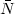0. These correlations are generally consistent with the hypothesis that the mass of intracluster gas increases with the virial mass of the cluster, and that the temperature of the gas increases with the velocity dispersion (depth of the cluster gravitational potential well). There is also an inverse correlation between Lx and the fraction of spirals in the cluster, which may reflect the stripping of spiral galaxies by the intracluster gas. All of these optical - X-ray correlations have natural explanations if the cluster X-ray emission is due to intracluster gas, but not if it is due to the other two mechanism discussed above.

The clearest evidence in favor of the thermal bremsstrahlung model is the detection of strong X-ray line emission from clusters (Sections 4.3.2 and 4.3.3). The strong 7 keV Fe line emission observed from clusters is very difficult to reconcile with any nonthermal model for the origin of the X-ray emission (Mitchell et al., 1976; Serlemitsos et al., 1977; Bahcall and Sarazin, 1977). This emission occurs naturally in the intracluster gas model if the abundances of heavy elements are roughly solar (see Section 5.2.2 below), while nonthermal emission processes would not directly produce any significant line emission (Sections 5.1.1 and 5.1.2). It is possible, of course, that the line emission arises from some different source than the generally distributed cluster X-ray emission; the line observations that have so far been made do not have sufficient spatial resolution to determine the location of the line emission region (Ulmer and Jernigan, 1978). However, the fact that the required abundance of iron is roughly constant from cluster to cluster (Section 4.3.2 and Figure 13) argues strongly against such a possibility. The clusters that have been observed differ widely in their properties, ranging from regular to irregular clusters, high X-ray luminosity to low luminosity clusters, clusters with strong radio sources to clusters without such sources, clusters with hard X-ray spectra to clusters with softer spectra, and so on. In fact, the strengths of the lines also vary widely; it is only the required abundances that are nearly constant. It is very unlikely that two independent sources for the line and continuum would vary simultaneously from cluster to cluster so as to maintain the appearance of a constant Fe abundance. Moreover, if the 7 keV Fe line were from a source that did not contribute significantly to the continuum X-ray emission, the iron abundance in this source would have to be vastly greater than solar. Such large iron abundances are certainly not common in astrophysical systems. Thus it appears very unlikely that the line emission and continuum emission could come from different sources; since the line emission is thermal, the continuum emission must be as well.

The nearly solar iron abundance in the intracluster gas, which has been derived from the 7 keV iron line, suggests that a significant portion of this gas has been processed in and ejected from stars. The total mass of X-ray emitting gas is very large, probably at least as large as the total mass in galaxies in a typical X-ray cluster (see Sec 4.4.1). Because of this large mass, the intracluster gas was initially believed to be primordial gas, which had never been bound in stars or even in galaxies (Gunn and Gott, 1972). During the formation of the universe (the big bang), hydrogen and helium were formed, but it is generally believed that no heavier elements could have been produced because of the lack of any stable isotopes with atomic weights of 5 or 8 (see, for example, Weinberg, 1972). The only sources which has been suggested for the formation of iron involve processing in stars. Moreover, the observed abundance in the intracluster gas is nearly the same as that in the solar system. The solar system is a second generation stellar system (the Sun is a Population I star). Thus it is possible that much of the intracluster gas has been processed through stars.

At the present epoch, there is no significant population of stars known that are not bound to galaxies. This may indicate that part of the intracluster gas may originally have been located within the galaxies. Alternatively, it is possible that there was a 'pregalactic' generation of stars (sometimes referred to as Population III stars; see, for example, Carr et al., 1984). Now, the mass of intracluster gas implied by the X-ray observations is nearly as large as the total mass of the galaxies in the cluster (see Section 4.4.1). If the intracluster gas did originate in part in galaxies, there has been a considerable exchange of gas between stars, galaxies, and the intracluster medium. Thus we are led by the X-ray line observations to a much more complicated picture of galaxy formation and evolution than we might have envisioned without this crucial piece of information. Theories of the origin of the intracluster gas and the formation of galaxies and clusters are discussed in more detail in Section 5.10.